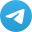I have encountered a curious problem at leetcode. You need to come up with a data structure that supports insertion, removing and retrieving a uniformly random element in average O(1) time. This problem is interesting because it shows how one can design data structures with amortized constant time complexity.

### std::vector reallocation strategy

I want to explain a little bit how std::vector reallocation works because I was inspired by it to design my solution to the problem which we will discuss later. It is well-known that the wors time of vector.push_back() operation os $$O(n)$$ if the maximum capacity is reached and it has to reallocate a new chunk of memory and copy elements there. But on average the complexity of a single push_back operation is $$O(1)$$.

Let’s prove it intuitively. Let’s imagine that a single CPU or memory operation like writing an integer in the allocated chunk of memory costs $1. Then we just need to show that we will pay only a linear amount of dollars for $$n$$ calls of push_back. Let’s pay$3 for every push_back. The vector will spend $1 to store a new element while maximal capacity is not reached and stash$2 for later. When the time comes to reallocate memory it has $$2n$$ dollars in the stash ($$2$$ per each element). Memory allocation is a very cheap operation on itself if we do no initialize it. After the vector reallocated a new chunk of memory twice larger than the previous one it spends $$n$$ dollars to copy elements in the new chunk.
So, we have just shown that for $$n$$ calls of push_back we had paid only a linear amount of money, which means that every operation is $$O(1)$$ on average.

A careful reader would ask why we paid $3 every push_back when$2 could be enough. There is one small detail that I deliberately omitted for simplicity. We prove by induction and after some reallocation happens the vector is half full and has \$0 in the stash. It means that until the next reallocation it has to save money not only for copying newly added elements but also the previous ones totaling in $$2n$$ elements.

### Problem statement

Design a data structure that supports all following operations in average $$O(1)$$ time. Note: Duplicate elements are allowed.

1. insert(val): Inserts an item val to the collection.
2. remove(val): Removes an item val from the collection if present.
3. getRandom(): Returns a random element from the current collection of elements. The probability of each element being returned is linearly related to the number of the same value the collection contains.

Example:

// Init an empty collection.
RandomizedCollection collection = new RandomizedCollection();

// Inserts 1 to the collection. Returns true as the collection did not contain 1.
collection.insert(1);

// Inserts another 1 to the collection. Returns false as the collection contained 1. Collection now contains [1,1].
collection.insert(1);

// Inserts 2 to the collection, returns true. Collection now contains [1,1,2].
collection.insert(2);

// getRandom should return 1 with the probability 2/3, and returns 2 with the probability 1/3.
collection.getRandom();

// Removes 1 from the collection, returns true. Collection now contains [1,2].
collection.remove(1);

// getRandom should return 1 and 2 both equally likely.
collection.getRandom();


The problem on the leetcode.

### Container Design

We store values of the inserted elements in a vector values. This will allow us to return a random uniform value from the available by a simple uniform sampling of the indices in a range $$[0, size - 1]$$.

To have $$O(1)$$ search and removing time we utilize a hash table (std::unordered_dict). Hash table stores pairs of keys and values. The key is the inserted number itself and the value in the table is the vector of indices of the inserted elements in the vector values. We can have several indices for the same number because duplicates are allowed.

Let us take a closer look at remove(val). To remove a value from our container we (i) find its positon in values by lookin-up in the hash table and set a flag REMOVED at this position; (ii) we remove the index of the removed value from the hast table as well. You can see that we do not explicitly erase an element from a vector because it is a linear-time operation in the worst case, we just flag that the value at that position is not valid.

If the number of cells flagged as REMOVED in the vector values becomes too large (i.e. $$> 1/2$$ of the vector size) we call method rebuild(). It erases all the REMOVED cells in a single pass through the vector. This operation is quite heavy. But similarly to the reallocation of the vector, which I discussed earlier, it can be shown that the amortized cost of a single remove() is constant.

Below I provide the full implementation of the container.

Feel free to ask me any questions in the comments below. Feedback is also very appreciated.

• Join my telegram channel@gradientdude
• Follow me on twitter@artsiom_s